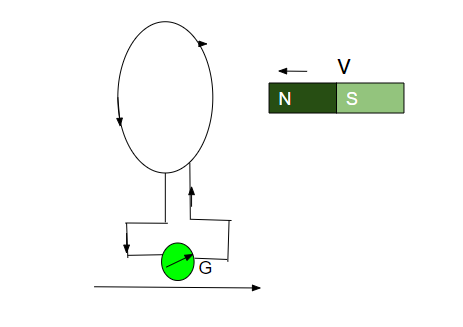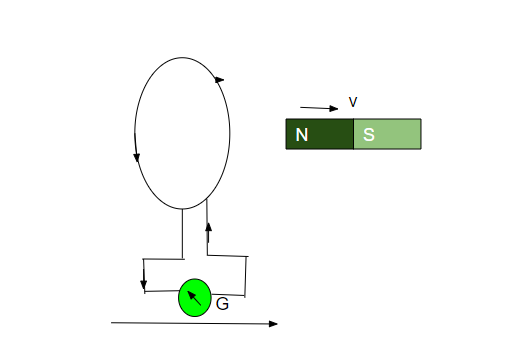Open in App
Not now

## Related Articles

• CBSE Class 12 Syllabus
• CBSE Class 12 Maths Notes
• CBSE Class 12 Physics Notes
• CBSE Class 12 Chemistry Notes
• CBSE Class 12 Accountancy Notes
• CBSE Class 12 Computer Science (Self-Paced Course)

# Lenz’s Law and Conservation of Energy

• Difficulty Level : Easy
• Last Updated : 19 Jul, 2022

While entering a mall/cinema hall/airport/ railway station you might face a security guard with a metal detector in his hand for a security checkup. You might have also used a credit/ debit card to pay the bills by swiping it into a machine called a card reader. You might have seen the AC generator also. All these works on the principle of Lenz’s law. We will talk about Lenz’s law in this article.

Electromagnetic induction: It is the phenomenon of production of induced emf due to change of magnetic flux (number of magnetic field lines) connect to a close circuit is called electromagnetic induction.

### What is Lenz’s law?

Lenz states that the direction of induced current in a circuit is such that it opposes the change in magnetic flux. Lenz’s is named after the German physicist “Emil Lenz “, who formulated it in 1834.

It is a scientific law that specifies the direction of induced current but states nothing about its magnitude. The magnetic flux connected to the closed-circuit amplifies the induced current flow in such a way that it creates a magnetic flux in the opposite direction of the original magnetic flux. The magnetic flux connected to a closed-circuit reduces the induced current flow in such a direction that magnetic flux is produced in the direction of the original current.

The Lenz’s Law formula is stated as,

E= -N(d∅/dt)

Here the negative sign indicates that the direction of induced emf is such that it opposes the change in magnetic flux).

Illustration 1:As shown in the figure above figure, When the North pole a bar magnet is moved towards the coil, the induced current in the coil flows in the anticlockwise direction, when we see it from the magnet side. The face of the coil develops north polarity. As we know, that same pole repels, so the north pole-north pole repels. So, it opposes the motion of the North pole of a magnet.

Conclusion: The motion of the magnet increases the flux through the coil and flux will be generated in the opposite direction by the induced current.

Illustration 2:As shown in the figure when the North pole of a bar magnet is taken away from the coil, the induced current in the coil flows in the clockwise direction. The face of the coil develops South polarity. We know that opposite poles attract. So, the north pole and south polarity attract towards each other.

Conclusion: The motion of the magnet decreases the flux through the coil. The flux is generated in the same direction by induced current, hence opposing and increasing the flux.

Important uses of Lenz’s law are:

• The braking system in trains
• AC generators
• Balancing Eddy current
• Metal detector
• Microphones
• Eddy’s current dynamic meter

### Lenz’s law and the Law of Conservation of Energy

Lenz’s law is a consequence of the law of conservation of energy. The law of conservation of energy states that energy can neither be created nor be destroyed, but it can be changed from one form to another form. Lenz’s law states that the direction of current is such that it opposes the change in the magnetic flux. So, an extra effort is required to do work against opposing forces. This extra work leads to periodic changes in magnetic flux hence more current is induced. Thus, the extra effort gets converted into electrical energy only, which is nothing but the law of conservation of energy.

The magnetic flux increases as the North Pole of the magnet approaches it and drops as it is driven away in the activity above. In the first scenario, opposing the cause involves moving the magnet, and the face facing the coil gains North Polarity. The magnet’s north pole and the coil’s north pole repel each other. To counteract the force of repulsion, mechanical action must be done to bring the magnet towards the coil. This mechanical energy is transformed into electrical energy. Due to Joule’s Effect, this electrical energy is turned into heat energy.

When the magnet is moved away from the coil, the coil’s nearer face obtains south polarity. In this instance, the produced emf will oppose the magnet’s outward motion. To resist the force of attraction between the North Pole of the magnet and the South Pole of the coil, mechanical labour must be done once more. This labour is transformed into electrical energy.

There is no mechanical work done if the magnet is not moved, hence no emf is induced in the coil.

As a result, Lenz’s Law is consistent with the law of conservation of energy.

### Faraday’s laws of electromagnetic induction:

First law: It states that whenever the magnetic flux is linked with a closed-circuit change, an emf is induced in it which lasts only as long as the change in flux is taking place. If the circuit is closed then current also gets induced inside the circuit which is called ” Induced current”.

Magnetic fields can be changed by:

• Moving a bar magnet towards or away from the coil.
• Moving the coil into the magnetic field or outside the magnetic field.
• Rotating the coil relative to the magnet.
• Changing the area of a coil placed in the magnetic field.

Second law: It states that the magnitude of the induced emf is equal to the rate of change of magnetic flux linked with the closed circuit.

|∈| = dⲫ/dt

E = -N dⲫ/dt

E = -N (ⲫ2-ⲫ1)/t ⇢ (” t “is time)

### Sample Questions

Question 1: What is the outcome of Lenz’s law?

Lenz’s law is a consequence of the law of conservation of energy.

Question 2: What will happen if Lenz’s law is reversed?

The induced current would produce a flux in the same direction at the original change.

Question 3: How is Lenz’s law used in real life?

Lenz’s law is used in electromagnetic brakes in trains. It is also applied in electric generator, AC generator.

Question 4: A closed-loop normally moves in a static electric field between the plates of a large capacitor. What is the amount of current produced when this capacitor is completely inside the region between the plates?

Current cannot be induced by changing electric flux. So, no current is produced when it is wholly inside the region between the capacitor plate.

Question 5: Difference between Faraday’s law and Lenz’s law?

Lenz’s law states the direction of an induced current.

Faraday’s law states that the magnitude of the emf induced in a circuit is proportional to the rate of change of magnetic flux.

Question 6: Which law is used in finding the direction of current in an AC generator?